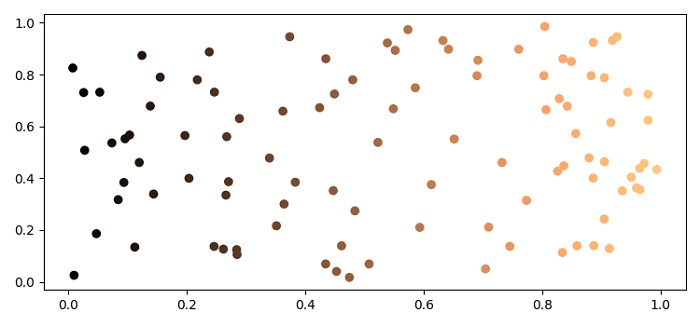# How to shade points in a scatter based on colormap in Matplotlib?

To shade points in a scatter based on colormap, we can use copper colormap in scatter() method.

## Steps

• Set the figure size and adjust the padding between and around the subplots.

• Create x and y random 100 data points using numpy.

• Plot scatter points x and y with color=x and colormap=copper.

• To display the figure, use show() method.

## Example

import numpy as np
from matplotlib import pyplot as plt, cm

plt.rcParams["figure.figsize"] = [7.50, 3.50]
plt.rcParams["figure.autolayout"] = True
x = np.random.rand(100)
y = np.random.rand(100)

plt.scatter(x, y, c=x, cmap='copper')

plt.show()

## Output